Courses

# Area Moments of Inertia Mechanical Engineering Notes | EduRev

## Mechanical Engineering : Area Moments of Inertia Mechanical Engineering Notes | EduRev

The document Area Moments of Inertia Mechanical Engineering Notes | EduRev is a part of the Mechanical Engineering Course Engineering Mechanics - Notes, Videos, MCQs & PPTs.
All you need of Mechanical Engineering at this link: Mechanical Engineering

Area Moment of inertia

The area moment of inertia is the second moment of area around a given axis. For example, given the axis O-O and the shaded area shown, one calculates the second moment of the area by adding together l2dA for all the elements of area dA in the shaded area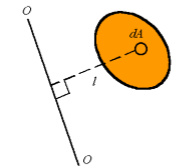The area moment of inertia, denoted by I, can, therefore, be calculated from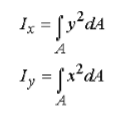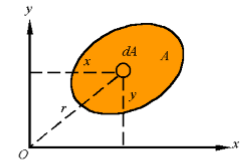The polar area moment of inertia, denoted by JO, is the area moment of inertia about the z-axis given by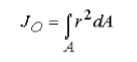Note that sinc r2=x2+y2 one has the relation

J0=Ix+Iy

The radius of gyration is the distance k away from the axis that all the area can be concentrated to result in the same moment of inertia. That is,

I=k2A

For a given area, one can define the radius of gyration around the x-axis, denoted by kx, the radius of gyration around the y-axis, denoted by ky, and the radius of gyration around the z-axis, denoted by kO. These are calculated from the relations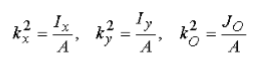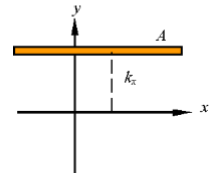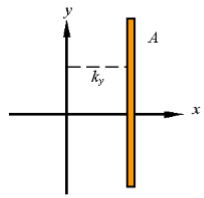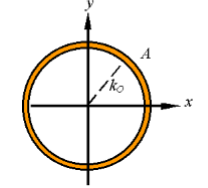It can easily to show from Jo=Ix+Iythat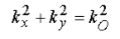The parallel axis theorem is a relation between the moment of inertia about an axis passing through the centroid and the moment of inertia about any parallel axis.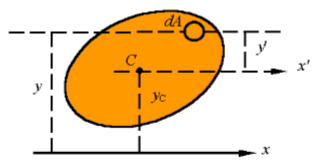Note that from the picture we have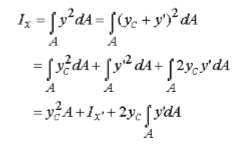Since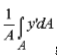gives the distance of the centroid above the x'-axis, and since the this distance is zero, one must conclude that the integral in the last term is zero so that the parallel axis theorem states that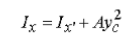where x' must pass through the centroid of the area. In this same way, one can show that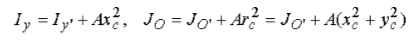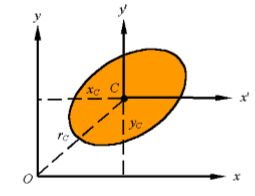In general, one can use the parallel axis theorem for any two parallel axes as long as one passes through the centroid. As shown in the picture, this is written as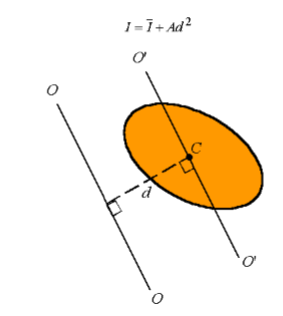where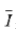is the moment of inertia about the axis O'-O' passing through the centroid, I is the moment of inertia about the axis O-O, and d is the perpendicular distance between the two parallel axis.

The moment of inertia of composite bodies can be calculated by adding together the moment of inertial of each of its sections. The only thing to remember is that all moments of inertia must be evaluated bout the same axis. Therefore, for example,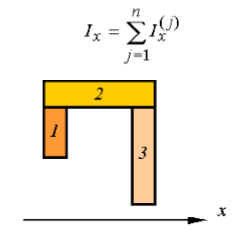To calculate the area moment of inertia of the composite body constructed of the three segments shown, one evaluates the moment of inertial of each part about the x-axis and adds the three together.

Offer running on EduRev: Apply code STAYHOME200 to get INR 200 off on our premium plan EduRev Infinity!

## Engineering Mechanics - Notes, Videos, MCQs & PPTs

30 videos|72 docs|65 tests

,

,

,

,

,

,

,

,

,

,

,

,

,

,

,

,

,

,

,

,

,

;